# Torque

Definition: Torque

Torque is the ability of a force to cause a body to rotate about a particular axis.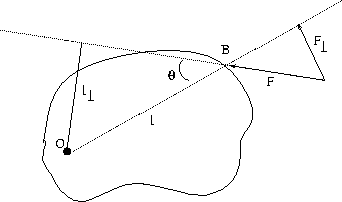In Figure 8.1, a force, F acts on an object at the point B . The object is pivoted so that it rotates about the point, O . The magnitude of the torquecaused by F about the axis through O is: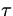= Fl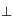where l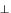(known as the moment arm of the force) is the perpendicular distance from the line of force to the axis through O :

l= lsinwhere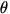is the angle between the line of force and the line OB .

Note:

• We can also write torque as= Fl= Flsin= Fl (1)

where F= Fsinis the component of F perpendicular to OB .
• The units of torque are Nm.
• Torque is defined to be positive if it tends to rotate the object counterclockwise, and negative if it tends to rotate the object clockwise.
• If an object is acted on by more than one force the total torque is the sum of the torques due to the individual forces: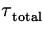=+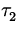+... (2)

where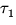= F1l1sin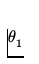, etc. Be sure to get the signs right for each individual torque.Next: Another Condition for Equilibrium Up: Rotational Equilibrium and Dynamics Previous: Rotational Equilibrium and Dynamics